1595054220

# Chapter 3 — Linear Regression From Scratch

In chapter 1 and chapter 2 , we got an introduction to PyTorch, some interesting functions used in PyTorch, different algorithms used in machine learning and a brief but solid introduction to linear regression. In this chapter we are going to build a Linear Regression model from scratch that is, without the use of any PyTorch built-ins. Without further ado, let’s begin.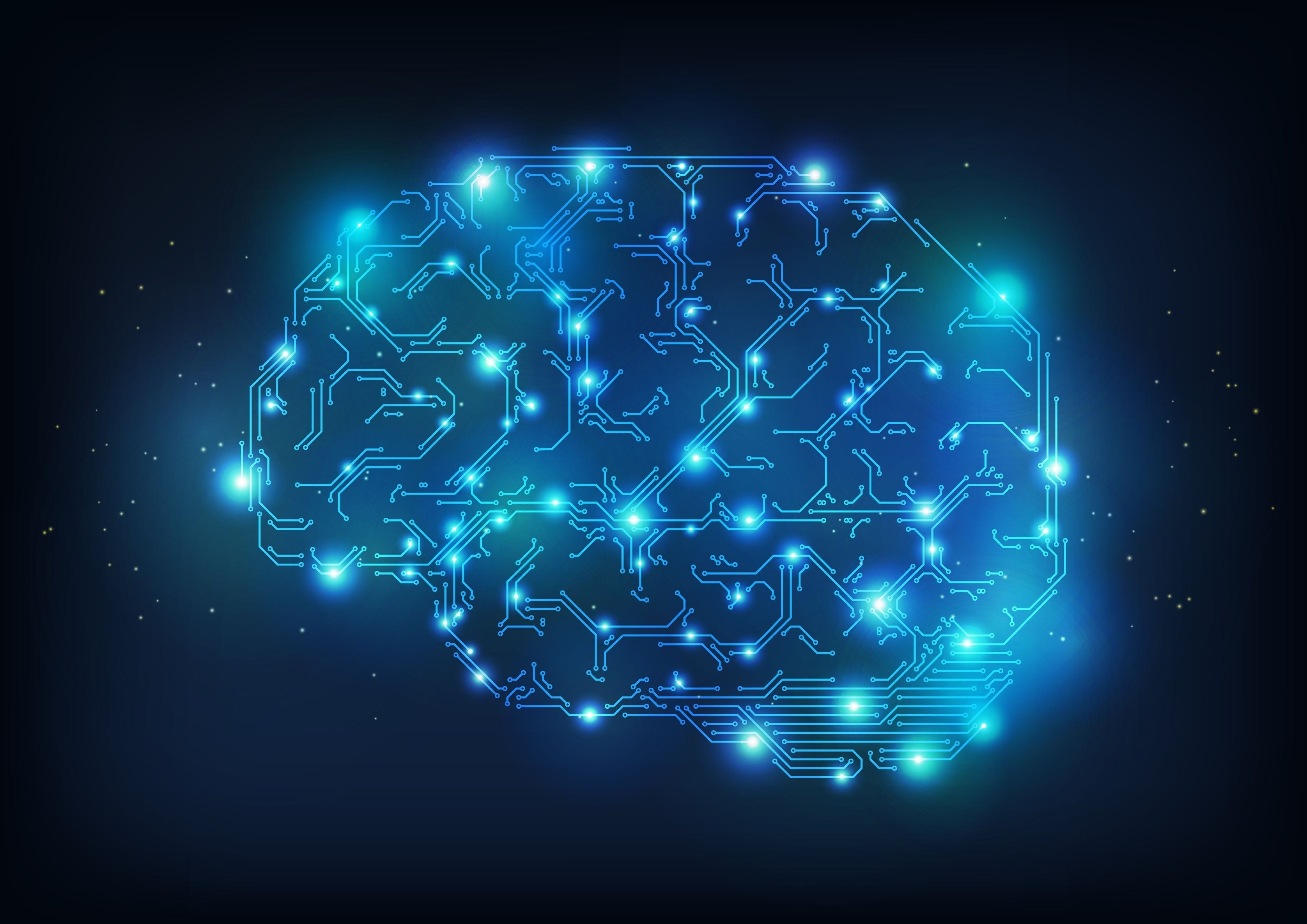## Problem Statement

Predict the yield of apples and oranges (dependent or target variables) by observing the average temperature, rainfall and humidity (independent or predictor variables ) for a region.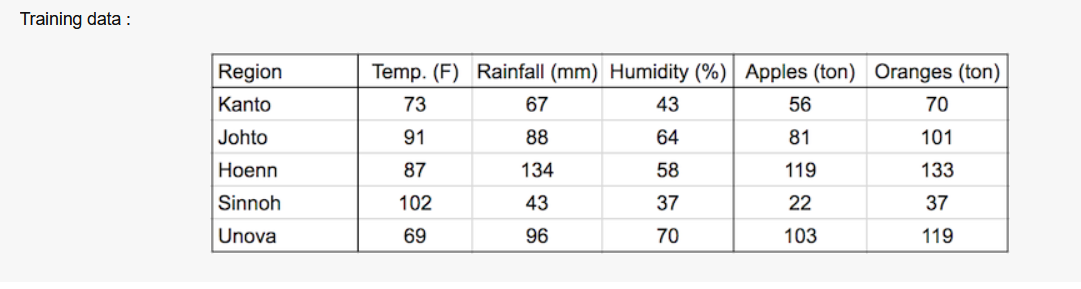We know that in linear regression target variables are considered as the weighted sum of independent variables, offset by some constant called bias (we add this offset so that when all the independent variables become zero the output should not become zero).

This statement can be represented mathematically as :-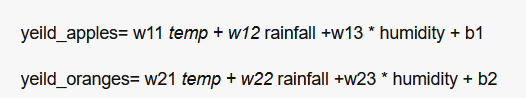These equations show that dependent variables share a linear relation with dependent variables.

## Learning Part of Linear Regression

We should find the set of weights and bias that is, w11,w12,…w23 and b1 and b2 by analyzing training data to predict the yield of apples and oranges of a new region based on avg. temp. , rainfall and humidity. This is done by adjusting the weights and biases slightly.

Training data can be considered as two numpy arrays (matrices), input and target one row per observation.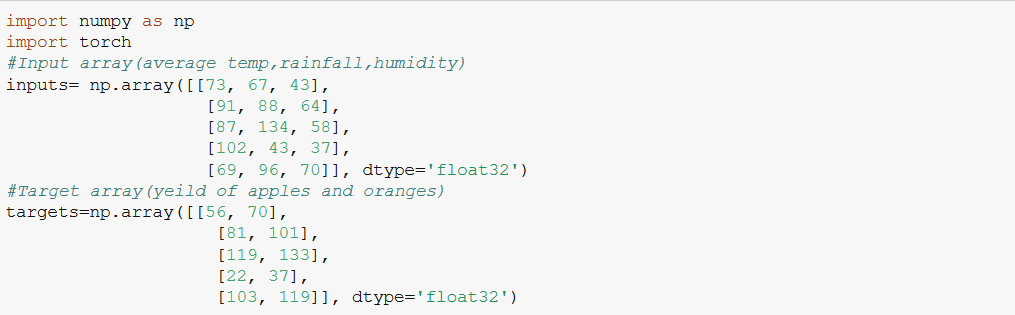Generated by Author

We now convert the numpy arrays to tensors for this purpose we use torch.from_numpy(), this method takes a numpy array as input and output tensor.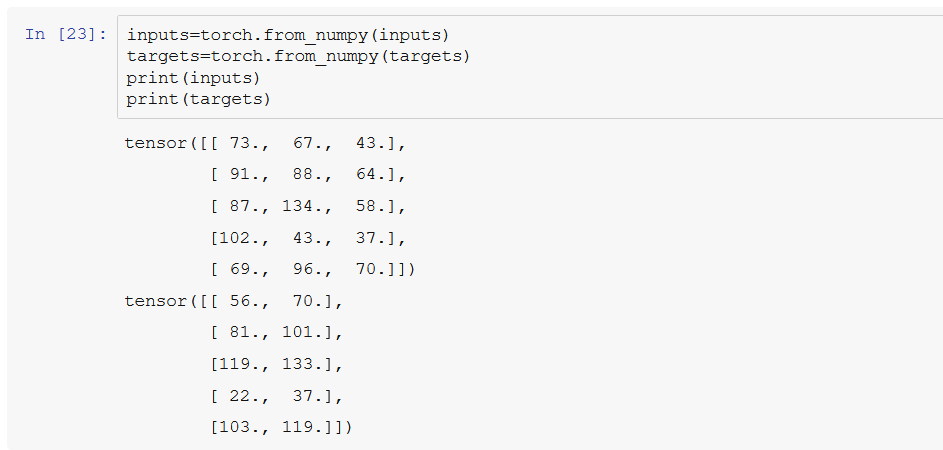Generated by Author

Weights (w11,w12,…w23) and biases (b1,b2) can be represented as matrices and first row and first bias element is used to predict the yield of apples and the second row and element the yield of oranges.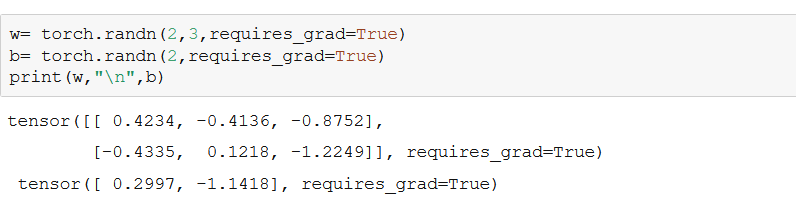Generated by Author

In this code snippet we create two tensors w and b using torch.randn() which will act as our weight and bias matrices.The torch.randn() function creates two matrices with element picked randomly from a normal distribution with mean=0 and standard deviation=1.

#deep-learning #linear-regression #scratch #tutorial #machine-learning #deep learning

## Buddha Community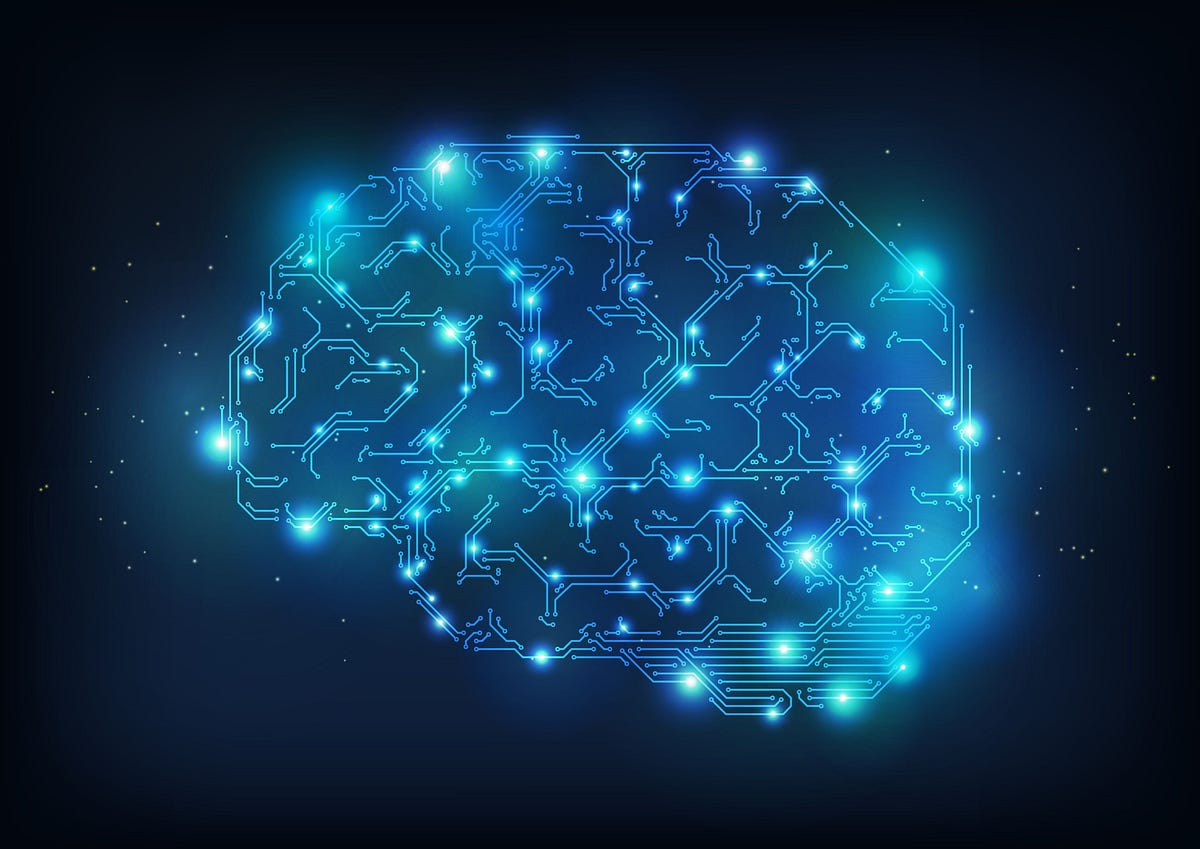1630743562

## A Wrapper for Sembast and SQFlite to Enable Easy

FHIR_DB

This is really just a wrapper around Sembast_SQFLite - so all of the heavy lifting was done by Alex Tekartik. I highly recommend that if you have any questions about working with this package that you take a look at Sembast. He's also just a super nice guy, and even answered a question for me when I was deciding which sembast version to use. As usual, ResoCoder also has a good tutorial.

I have an interest in low-resource settings and thus a specific reason to be able to store data offline. To encourage this use, there are a number of other packages I have created based around the data format FHIR. FHIR® is the registered trademark of HL7 and is used with the permission of HL7. Use of the FHIR trademark does not constitute endorsement of this product by HL7.

## Using the Db

So, while not absolutely necessary, I highly recommend that you use some sort of interface class. This adds the benefit of more easily handling errors, plus if you change to a different database in the future, you don't have to change the rest of your app, just the interface.

I've used something like this in my projects:

``````class IFhirDb {
IFhirDb();
final ResourceDao resourceDao = ResourceDao();

Future<Either<DbFailure, Resource>> save(Resource resource) async {
Resource resultResource;
try {
resultResource = await resourceDao.save(resource);
} catch (error) {
return left(DbFailure.unableToSave(error: error.toString()));
}
return right(resultResource);
}

Future<Either<DbFailure, List<Resource>>> returnListOfSingleResourceType(
String resourceType) async {
List<Resource> resultList;
try {
resultList =
await resourceDao.getAllSortedById(resourceType: resourceType);
} catch (error) {
return left(DbFailure.unableToObtainList(error: error.toString()));
}
return right(resultList);
}

Future<Either<DbFailure, List<Resource>>> searchFunction(
String resourceType, String searchString, String reference) async {
List<Resource> resultList;
try {
resultList =
await resourceDao.searchFor(resourceType, searchString, reference);
} catch (error) {
return left(DbFailure.unableToObtainList(error: error.toString()));
}
return right(resultList);
}
}
``````

I like this because in case there's an i/o error or something, it won't crash your app. Then, you can call this interface in your app like the following:

``````final patient = Patient(
resourceType: 'Patient',
name: [HumanName(text: 'New Patient Name')],
birthDate: Date(DateTime.now()),
);

final saveResult = await IFhirDb().save(patient);
``````

This will save your newly created patient to the locally embedded database.

IMPORTANT: this database will expect that all previously created resources have an id. When you save a resource, it will check to see if that resource type has already been stored. (Each resource type is saved in it's own store in the database). It will then check if there is an ID. If there's no ID, it will create a new one for that resource (along with metadata on version number and creation time). It will save it, and return the resource. If it already has an ID, it will copy the the old version of the resource into a _history store. It will then update the metadata of the new resource and save that version into the appropriate store for that resource. If, for instance, we have a previously created patient:

``````{
"resourceType": "Patient",
"id": "fhirfli-294057507-6811107",
"meta": {
"versionId": "1",
"lastUpdated": "2020-10-16T19:41:28.054369Z"
},
"name": [
{
"given": ["New"],
"family": "Patient"
}
],
"birthDate": "2020-10-16"
}
``````

And we update the last name to 'Provider'. The above version of the patient will be kept in _history, while in the 'Patient' store in the db, we will have the updated version:

``````{
"resourceType": "Patient",
"id": "fhirfli-294057507-6811107",
"meta": {
"versionId": "2",
"lastUpdated": "2020-10-16T19:45:07.316698Z"
},
"name": [
{
"given": ["New"],
"family": "Provider"
}
],
"birthDate": "2020-10-16"
}
``````

This way we can keep track of all previous version of all resources (which is obviously important in medicine).

For most of the interactions (saving, deleting, etc), they work the way you'd expect. The only difference is search. Because Sembast is NoSQL, we can search on any of the fields in a resource. If in our interface class, we have the following function:

``````  Future<Either<DbFailure, List<Resource>>> searchFunction(
String resourceType, String searchString, String reference) async {
List<Resource> resultList;
try {
resultList =
await resourceDao.searchFor(resourceType, searchString, reference);
} catch (error) {
return left(DbFailure.unableToObtainList(error: error.toString()));
}
return right(resultList);
}
``````

You can search for all immunizations of a certain patient:

``````searchFunction(
'Immunization', 'patient.reference', 'Patient/\$patientId');
``````

This function will search through all entries in the 'Immunization' store. It will look at all 'patient.reference' fields, and return any that match 'Patient/\$patientId'.

The last thing I'll mention is that this is a password protected db, using AES-256 encryption (although it can also use Salsa20). Anytime you use the db, you have the option of using a password for encryption/decryption. Remember, if you setup the database using encryption, you will only be able to access it using that same password. When you're ready to change the password, you will need to call the update password function. If we again assume we created a change password method in our interface, it might look something like this:

``````class IFhirDb {
IFhirDb();
final ResourceDao resourceDao = ResourceDao();
...
try {
} catch (error) {
}
return right(Unit);
}
``````

You don't have to use a password, and in that case, it will save the db file as plain text. If you want to add a password later, it will encrypt it at that time.

### General Store

After using this for a while in an app, I've realized that it needs to be able to store data apart from just FHIR resources, at least on occasion. For this, I've added a second class for all versions of the database called GeneralDao. This is similar to the ResourceDao, but fewer options. So, in order to save something, it would look like this:

``````await GeneralDao().save('password', {'new':'map'});
``````

The difference between these two options is that the first one will generate a key for the map being stored, while the second will store the map using the key provided. Both will return the key after successfully storing the map.

Other functions available include:

``````// deletes everything in the general store

// delete specific entry

// returns map with that key
``````

FHIR® is a registered trademark of Health Level Seven International (HL7) and its use does not constitute an endorsement of products by HL7®

## Use this package as a library

### Depend on it

Run this command:

With Flutter:

`` \$ flutter pub add fhir_db``

This will add a line like this to your package's pubspec.yaml (and run an implicit flutter pub get):

``````dependencies:
fhir_db: ^0.4.3``````

### Import it

Now in your Dart code, you can use:

``````import 'package:fhir_db/dstu2.dart';
import 'package:fhir_db/dstu2/fhir_db.dart';
import 'package:fhir_db/dstu2/general_dao.dart';
import 'package:fhir_db/dstu2/resource_dao.dart';
import 'package:fhir_db/encrypt/aes.dart';
import 'package:fhir_db/encrypt/salsa.dart';
import 'package:fhir_db/r4.dart';
import 'package:fhir_db/r4/fhir_db.dart';
import 'package:fhir_db/r4/general_dao.dart';
import 'package:fhir_db/r4/resource_dao.dart';
import 'package:fhir_db/r5.dart';
import 'package:fhir_db/r5/fhir_db.dart';
import 'package:fhir_db/r5/general_dao.dart';
import 'package:fhir_db/r5/resource_dao.dart';
import 'package:fhir_db/stu3.dart';
import 'package:fhir_db/stu3/fhir_db.dart';
import 'package:fhir_db/stu3/general_dao.dart';
import 'package:fhir_db/stu3/resource_dao.dart'; ``````

example/lib/main.dart

``````import 'package:fhir/r4.dart';
import 'package:fhir_db/r4.dart';
import 'package:flutter/material.dart';
import 'package:test/test.dart';

Future<void> main() async {
WidgetsFlutterBinding.ensureInitialized();

final resourceDao = ResourceDao();

// await resourceDao.updatePw('newPw', null);
await resourceDao.deleteAllResources(null);

test('Playing with Passwords', () async {
final patient = Patient(id: Id('1'));

final saved = await resourceDao.save(null, patient);

await resourceDao.updatePw(null, 'newPw');
final search1 = await resourceDao.find('newPw',
resourceType: R4ResourceType.Patient, id: Id('1'));
expect(saved, search1);

resourceType: R4ResourceType.Patient, id: Id('1'));
expect(saved, search2);

final search3 = await resourceDao.find(null,
resourceType: R4ResourceType.Patient, id: Id('1'));
expect(saved, search3);

await resourceDao.deleteAllResources(null);
});
});

final id = Id('12345');
group('Saving Things:', () {
test('Save Patient', () async {
final humanName = HumanName(family: 'Atreides', given: ['Duke']);
final patient = Patient(id: id, name: [humanName]);
final saved = await resourceDao.save(null, patient);

expect(saved.id, id);

expect((saved as Patient).name?, humanName);
});

test('Save Organization', () async {
final organization = Organization(id: id, name: 'FhirFli');
final saved = await resourceDao.save(null, organization);

expect(saved.id, id);

expect((saved as Organization).name, 'FhirFli');
});

test('Save Observation1', () async {
final observation1 = Observation(
id: Id('obs1'),
code: CodeableConcept(text: 'Observation #1'),
effectiveDateTime: FhirDateTime(DateTime(1981, 09, 18)),
);
final saved = await resourceDao.save(null, observation1);

expect(saved.id, Id('obs1'));

expect((saved as Observation).code.text, 'Observation #1');
});

test('Save Observation1 Again', () async {
final observation1 = Observation(
id: Id('obs1'),
code: CodeableConcept(text: 'Observation #1 - Updated'));
final saved = await resourceDao.save(null, observation1);

expect(saved.id, Id('obs1'));

expect((saved as Observation).code.text, 'Observation #1 - Updated');

expect(saved.meta?.versionId, Id('2'));
});

test('Save Observation2', () async {
final observation2 = Observation(
id: Id('obs2'),
code: CodeableConcept(text: 'Observation #2'),
effectiveDateTime: FhirDateTime(DateTime(1981, 09, 18)),
);
final saved = await resourceDao.save(null, observation2);

expect(saved.id, Id('obs2'));

expect((saved as Observation).code.text, 'Observation #2');
});

test('Save Observation3', () async {
final observation3 = Observation(
id: Id('obs3'),
code: CodeableConcept(text: 'Observation #3'),
effectiveDateTime: FhirDateTime(DateTime(1981, 09, 18)),
);
final saved = await resourceDao.save(null, observation3);

expect(saved.id, Id('obs3'));

expect((saved as Observation).code.text, 'Observation #3');
});
});

group('Finding Things:', () {
test('Find 1st Patient', () async {
final search = await resourceDao.find(null,
resourceType: R4ResourceType.Patient, id: id);
final humanName = HumanName(family: 'Atreides', given: ['Duke']);

expect(search.length, 1);

expect((search as Patient).name?, humanName);
});

test('Find 3rd Observation', () async {
final search = await resourceDao.find(null,
resourceType: R4ResourceType.Observation, id: Id('obs3'));

expect(search.length, 1);

expect(search.id, Id('obs3'));

expect((search as Observation).code.text, 'Observation #3');
});

test('Find All Observations', () async {
final search = await resourceDao.getResourceType(
null,
resourceTypes: [R4ResourceType.Observation],
);

expect(search.length, 3);

final idList = [];
for (final obs in search) {
}

expect(idList.contains('obs1'), true);

expect(idList.contains('obs2'), true);

expect(idList.contains('obs3'), true);
});

test('Find All (non-historical) Resources', () async {
final search = await resourceDao.getAll(null);

expect(search.length, 5);
final patList = search.toList();
final orgList = search.toList();
final obsList = search.toList();
patList.retainWhere(
(resource) => resource.resourceType == R4ResourceType.Patient);
orgList.retainWhere(
(resource) => resource.resourceType == R4ResourceType.Organization);
obsList.retainWhere(
(resource) => resource.resourceType == R4ResourceType.Observation);

expect(patList.length, 1);

expect(orgList.length, 1);

expect(obsList.length, 3);
});
});

group('Deleting Things:', () {
test('Delete 2nd Observation', () async {
await resourceDao.delete(
null, null, R4ResourceType.Observation, Id('obs2'), null, null);

final search = await resourceDao.getResourceType(
null,
resourceTypes: [R4ResourceType.Observation],
);

expect(search.length, 2);

final idList = [];
for (final obs in search) {
}

expect(idList.contains('obs1'), true);

expect(idList.contains('obs2'), false);

expect(idList.contains('obs3'), true);
});

test('Delete All Observations', () async {
await resourceDao.deleteSingleType(null,
resourceType: R4ResourceType.Observation);

final search = await resourceDao.getAll(null);

expect(search.length, 2);

final patList = search.toList();
final orgList = search.toList();
patList.retainWhere(
(resource) => resource.resourceType == R4ResourceType.Patient);
orgList.retainWhere(
(resource) => resource.resourceType == R4ResourceType.Organization);

expect(patList.length, 1);

expect(patList.length, 1);
});

test('Delete All Resources', () async {
await resourceDao.deleteAllResources(null);

final search = await resourceDao.getAll(null);

expect(search.length, 0);
});
});

group('Password - Saving Things:', () {
test('Save Patient', () async {
await resourceDao.updatePw(null, 'newPw');
final humanName = HumanName(family: 'Atreides', given: ['Duke']);
final patient = Patient(id: id, name: [humanName]);
final saved = await resourceDao.save('newPw', patient);

expect(saved.id, id);

expect((saved as Patient).name?, humanName);
});

test('Save Organization', () async {
final organization = Organization(id: id, name: 'FhirFli');
final saved = await resourceDao.save('newPw', organization);

expect(saved.id, id);

expect((saved as Organization).name, 'FhirFli');
});

test('Save Observation1', () async {
final observation1 = Observation(
id: Id('obs1'),
code: CodeableConcept(text: 'Observation #1'),
effectiveDateTime: FhirDateTime(DateTime(1981, 09, 18)),
);
final saved = await resourceDao.save('newPw', observation1);

expect(saved.id, Id('obs1'));

expect((saved as Observation).code.text, 'Observation #1');
});

test('Save Observation1 Again', () async {
final observation1 = Observation(
id: Id('obs1'),
code: CodeableConcept(text: 'Observation #1 - Updated'));
final saved = await resourceDao.save('newPw', observation1);

expect(saved.id, Id('obs1'));

expect((saved as Observation).code.text, 'Observation #1 - Updated');

expect(saved.meta?.versionId, Id('2'));
});

test('Save Observation2', () async {
final observation2 = Observation(
id: Id('obs2'),
code: CodeableConcept(text: 'Observation #2'),
effectiveDateTime: FhirDateTime(DateTime(1981, 09, 18)),
);
final saved = await resourceDao.save('newPw', observation2);

expect(saved.id, Id('obs2'));

expect((saved as Observation).code.text, 'Observation #2');
});

test('Save Observation3', () async {
final observation3 = Observation(
id: Id('obs3'),
code: CodeableConcept(text: 'Observation #3'),
effectiveDateTime: FhirDateTime(DateTime(1981, 09, 18)),
);
final saved = await resourceDao.save('newPw', observation3);

expect(saved.id, Id('obs3'));

expect((saved as Observation).code.text, 'Observation #3');
});
});

group('Password - Finding Things:', () {
test('Find 1st Patient', () async {
final search = await resourceDao.find('newPw',
resourceType: R4ResourceType.Patient, id: id);
final humanName = HumanName(family: 'Atreides', given: ['Duke']);

expect(search.length, 1);

expect((search as Patient).name?, humanName);
});

test('Find 3rd Observation', () async {
final search = await resourceDao.find('newPw',
resourceType: R4ResourceType.Observation, id: Id('obs3'));

expect(search.length, 1);

expect(search.id, Id('obs3'));

expect((search as Observation).code.text, 'Observation #3');
});

test('Find All Observations', () async {
final search = await resourceDao.getResourceType(
'newPw',
resourceTypes: [R4ResourceType.Observation],
);

expect(search.length, 3);

final idList = [];
for (final obs in search) {
}

expect(idList.contains('obs1'), true);

expect(idList.contains('obs2'), true);

expect(idList.contains('obs3'), true);
});

test('Find All (non-historical) Resources', () async {
final search = await resourceDao.getAll('newPw');

expect(search.length, 5);
final patList = search.toList();
final orgList = search.toList();
final obsList = search.toList();
patList.retainWhere(
(resource) => resource.resourceType == R4ResourceType.Patient);
orgList.retainWhere(
(resource) => resource.resourceType == R4ResourceType.Organization);
obsList.retainWhere(
(resource) => resource.resourceType == R4ResourceType.Observation);

expect(patList.length, 1);

expect(orgList.length, 1);

expect(obsList.length, 3);
});
});

group('Password - Deleting Things:', () {
test('Delete 2nd Observation', () async {
await resourceDao.delete(
'newPw', null, R4ResourceType.Observation, Id('obs2'), null, null);

final search = await resourceDao.getResourceType(
'newPw',
resourceTypes: [R4ResourceType.Observation],
);

expect(search.length, 2);

final idList = [];
for (final obs in search) {
}

expect(idList.contains('obs1'), true);

expect(idList.contains('obs2'), false);

expect(idList.contains('obs3'), true);
});

test('Delete All Observations', () async {
await resourceDao.deleteSingleType('newPw',
resourceType: R4ResourceType.Observation);

final search = await resourceDao.getAll('newPw');

expect(search.length, 2);

final patList = search.toList();
final orgList = search.toList();
patList.retainWhere(
(resource) => resource.resourceType == R4ResourceType.Patient);
orgList.retainWhere(
(resource) => resource.resourceType == R4ResourceType.Organization);

expect(patList.length, 1);

expect(patList.length, 1);
});

test('Delete All Resources', () async {
await resourceDao.deleteAllResources('newPw');

final search = await resourceDao.getAll('newPw');

expect(search.length, 0);

await resourceDao.updatePw('newPw', null);
});
});
} ``````

Author: MayJuun1595054220

## Chapter 3 — Linear Regression From Scratch

In chapter 1 and chapter 2 , we got an introduction to PyTorch, some interesting functions used in PyTorch, different algorithms used in machine learning and a brief but solid introduction to linear regression. In this chapter we are going to build a Linear Regression model from scratch that is, without the use of any PyTorch built-ins. Without further ado, let’s begin.## Problem Statement

Predict the yield of apples and oranges (dependent or target variables) by observing the average temperature, rainfall and humidity (independent or predictor variables ) for a region.We know that in linear regression target variables are considered as the weighted sum of independent variables, offset by some constant called bias (we add this offset so that when all the independent variables become zero the output should not become zero).

This statement can be represented mathematically as :-These equations show that dependent variables share a linear relation with dependent variables.

## Learning Part of Linear Regression

We should find the set of weights and bias that is, w11,w12,…w23 and b1 and b2 by analyzing training data to predict the yield of apples and oranges of a new region based on avg. temp. , rainfall and humidity. This is done by adjusting the weights and biases slightly.

Training data can be considered as two numpy arrays (matrices), input and target one row per observation.Generated by Author

We now convert the numpy arrays to tensors for this purpose we use torch.from_numpy(), this method takes a numpy array as input and output tensor.Generated by Author

Weights (w11,w12,…w23) and biases (b1,b2) can be represented as matrices and first row and first bias element is used to predict the yield of apples and the second row and element the yield of oranges.Generated by Author

In this code snippet we create two tensors w and b using torch.randn() which will act as our weight and bias matrices.The torch.randn() function creates two matrices with element picked randomly from a normal distribution with mean=0 and standard deviation=1.

#deep-learning #linear-regression #scratch #tutorial #machine-learning #deep learning1594271340

## A Deep Dive into Linear Regression

Let’s begin our journey with the truth — machines never learn. What a typical machine learning algorithm does is find a mathematical equation that, when applied to a given set of training data, produces a prediction that is very close to the actual output.

Why is this not learning? Because if you change the training data or environment even slightly, the algorithm will go haywire! Not how learning works in humans. If you learned to play a video game by looking straight at the screen, you would still be a good player if the screen is slightly tilted by someone, which would not be the case in ML algorithms.

However, most of the algorithms are so complex and intimidating that it gives our mere human intelligence the feel of actual learning, effectively hiding the underlying math within. There goes a dictum that if you can implement the algorithm, you know the algorithm. This saying is lost in the dense jungle of libraries and inbuilt modules which programming languages provide, reducing us to regular programmers calling an API and strengthening further this notion of a black box. Our quest will be to unravel the mysteries of this so-called ‘black box’ which magically produces accurate predictions, detects objects, diagnoses diseases and claims to surpass human intelligence one day.

We will start with one of the not-so-complex and easy to visualize algorithm in the ML paradigm — Linear Regression. The article is divided into the following sections:

1. Need for Linear Regression

2. Visualizing Linear Regression

3. Deriving the formula for weight matrix W

4. Using the formula and performing linear regression on a real world data set

Note: Knowledge on Linear Algebra, a little bit of Calculus and Matrices are a prerequisite to understanding this article

Also, a basic understanding of python, NumPy, and Matplotlib are a must.

## 1) Need for Linear regression

Regression means predicting a real valued number from a given set of input variables. Eg. Predicting temperature based on month of the year, humidity, altitude above sea level, etc. Linear Regression would therefore mean predicting a real valued number that follows a linear trend. Linear regression is the first line of attack to discover correlations in our data.

Now, the first thing that comes to our mind when we hear the word linear is, a line.

Yes! In linear regression, we try to fit a line that best generalizes all the data points in the data set. By generalizing, we mean we try to fit a line that passes very close to all the data points.

But how do we ensure that this happens? To understand this, let’s visualize a 1-D Linear Regression. This is also called as Simple Linear Regression

#calculus #machine-learning #linear-regression-math #linear-regression #linear-regression-python #python1592023980

## 5 Regression algorithms: Explanation & Implementation in Python

Take your current understanding and skills on machine learning algorithms to the next level with this article. What is regression analysis in simple words? How is it applied in practice for real-world problems? And what is the possible snippet of codes in Python you can use for implementation regression algorithms for various objectives? Let’s forget about boring learning stuff and talk about science and the way it works.

#linear-regression-python #linear-regression #multivariate-regression #regression #python-programming1598352300

## Regression: Linear Regression

Machine learning algorithms are not your regular algorithms that we may be used to because they are often described by a combination of some complex statistics and mathematics. Since it is very important to understand the background of any algorithm you want to implement, this could pose a challenge to people with a non-mathematical background as the maths can sap your motivation by slowing you down.In this article, we would be discussing linear and logistic regression and some regression techniques assuming we all have heard or even learnt about the Linear model in Mathematics class at high school. Hopefully, at the end of the article, the concept would be clearer.

**Regression Analysis **is a statistical process for estimating the relationships between the dependent variables (say Y) and one or more independent variables or predictors (X). It explains the changes in the dependent variables with respect to changes in select predictors. Some major uses for regression analysis are in determining the strength of predictors, forecasting an effect, and trend forecasting. It finds the significant relationship between variables and the impact of predictors on dependent variables. In regression, we fit a curve/line (regression/best fit line) to the data points, such that the differences between the distances of data points from the curve/line are minimized.

#regression #machine-learning #beginner #logistic-regression #linear-regression #deep learning